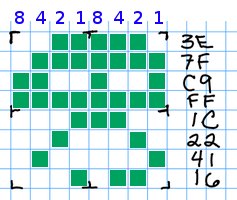# Quiz 2 Solutions

Wed Feb 11

You have up to 20 minutes. You may use a standard calculator if necessary, but no text book or notes.

1. +5 Below is a tree representing a variable-width encoding of 9 letters. Use it to:

• decode the bits 10011111010011010 into a word: WHINE
• encode the word WITH as bits: 1001101011112. +5 Decode the following 8×8 image indicated by the hexadecimal number along the right side, which uses 1 bit per pixel.3. +5 If an image uses 9 bits for each pixel, what is the maximum number of distinct colors it can contain?

$$2^9 = 512$$ colors

4. +5 Draw a tree representing a variable-width encoding of the four letters A, L, N, and T. Use it to encode the word ATLANTA. The fixed-width tree (below) uses exactly 2 bits per character, so encoding ATLANTA requires 14 bits. How many bits does your tree need to encode ATLANTA?A fixed-width encoding of four letters.

Here is one possible solution. In this tree, L and N are extended to 3 bits each, so that A can be just 1 bit. We can then encode ATLANTA as 13 bits.